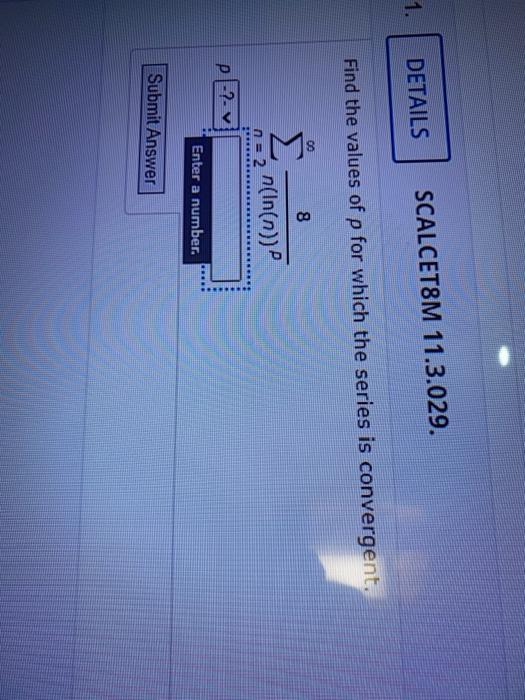### Create an Account

Home / Questions / DETAILS SCALCET8M 11.3.029. Find the values of p for which the series is convergent. 00 8 ...

# DETAILS SCALCET8M 11.3.029. Find the values of p for which the series is convergent. 00 8 n = 2 n(In(n)) P?. V Enter a number. Submit Answer

DETAILS SCALCET8M 11.3.029. Find the values of p for which the series is convergent. 00 8 n = 2 n(In(n)) P?. V Enter a number. Submit AnswerJun 03 2021 View more View LessSubscribe To Get Solution